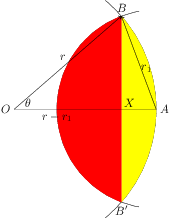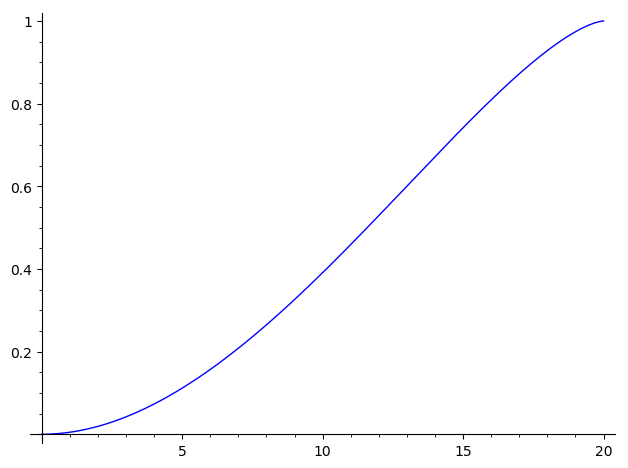# A goat in a tether

I would like to solve the following goat problem in sage.

A goat is on the tether on the boundary of the circle with radius 10. What is the radius of the tether is the goat manages to eat half of the grass?

I managed to solve it by Python when I derived the equation.

import numpy
import scipy
import scipy.optimize
def f(x):
y=400*x*numpy.cos(x)**2-100*numpy.sin(2*x)-200*x+50*numpy.pi
return y

angle = scipy.optimize.newton(f, 1)
print(20*numpy.cos(angle))


But I was wondering if this can be solved by sage such that I make equations for circle with center (0,0) and radius 10 and circle with center (0,1) and radius x_1, compute the intersections numerically, find the area between curves numerically and iterate this for different radius x_n until the suitable accuracy is found.

The problem, I found is that I don't know how to fund numerically the coordinates of the intersections of two circles and pass them to the numerical integrator.

edit retag close merge delete

No answer to my previous question ? My hunch might have been right... Nevertheless, I'll throw a couple bones at you :

• your problem has an analytic/geometric solution (high school level).
• You can also write a numerical integral solution much more simply. (think double integration)...
1

Actually it was not homework. I just wanted to learn Sagemath to do numerical integration.

Sort by » oldest newest most voted

Okay. After 12 days, your (hypothetic) homework is past due. Let's look at a couple of solutions.

"when you're lost, draw what you know." Undergrads' wisdom. Let's see:

Without loss of generality, set up a framework placing the fence center in $O=(0,0)$ and the tether anchor in $A=(r_0,0)$. In this framework a point $(x,y)$ is edible by the goat if it is :

• inside the fence, implying $x^2+y^2<=r_0^2$, and
• closer to the tether's anchor than $r_1$, implying $(r-x)^2+y^2<=r1^2$.

In other words, the edible region is the intersection of two disks $D_0$, centered in $O$ with radius $r_0$ and $D_1$, centered in $A$ with radius $r_1$. This region can be exactly partitioned by the straight line segment joining the intersections of the two circles boundaries of these disks:Each of these regions is the intersection of a disk of radius $r$ and a half-plane cutting the disk at a distance $X<=r$ of its center.

Therefore, a first, (almost) purely geometrical, solution to your problem is to derive the area of such a surface, and apply it to both the "yellow" and the "red" surfaces. Let's consider the "yellow" one, delimited bu th arc $B'AB$ and the straight segment $BB'$. This surface is symmetric about $OA$ ; therefore, its area is twice of the surface bounded by the arc $AB$ and the straight segments $BX$ and $XA$.

This latter surface can be in turn considered as the difference between

• the circular sector delimited by$OA$, $OB$, and
• the arc $AB$ and the triangle $BOX$.

Let's call $\theta$ the angle $\widehat{AOB}$. It can be determined from the position of $B$. We have of course $\cos\theta=\frac{OX}{r}=\frac{B_x}{r}$ and $\tan\theta={B_y}{B_x}$. At the high school level, this "determination" is a bit fuzzy, but will be clarified in first year of college...

The circular sector has area $\frac{r^2\theta}{2}$, the triangle has area $\frac{OX*XB}{2}=\frac{B_x B_y}{2}$. The yellow surface has area twice their difference, therefore $r_0^2 \theta - B_x\cdot B_y$.

For similar reasons (left to the reader as an exercise ; beware the signs !), with $\cos\theta_1=\frac{B_y}{r_1}$, the "red" sector has area $r_1^2\theta_1-(r_0-B_x) B_y$.

All that is lacking are the coordinates of $B$ which are determined by the fact that it belongs to both the boundary circles (the fence and the tether). Since there are two solution points, we'll select the one with positive ordinate :

x,y,r_0,r_1=var("x,y,r_0,r_1")
assume(x,"real",y,"real",r_0>0,r_1>0,r_1<2*r_0)
O=vector([0,0])
A=vector([r_0,0])
b=vector([x,y])
E0=(b-O).norm()^2==r_0^2
E1=(b-A).norm()^2==r_1^2
Sol=solve([E0, E1],[x,y], solution_dict=True)
if bool(Sol.get(y)>0):
B=vector([Sol.get(x),Sol.get(y)])
else:
B=vector([Sol.get(x),Sol.get(y)])
X=B
Y=B


That gives us $B=\left(\frac{2 \, r_{0}^{2} - r_{1}^{2}}{2 \, r_{0}},\ \frac{\sqrt{4 \, r_{0}^{2} - r_{1}^{2}} r_{1}}{2 \, r_{0}}\right)$.

This also determines the angle $\theta=\widehat{AOB}$ by either theta=arccos(X/r_0) or theta=arctan2(Y,X) (Note : the third "obvious" choice, theta=arcsin(Y./r_0 is unsuitable here, given the choice of branches of Sage's implementation of arcsin). We determine $\theta_1$ in a similar way (again, mind the signs...).

Summing the partial areas and finding the root (value of $r_1$ leaving an edible area of 10 when $r_0=10$) is left as an exercise to the reader...

This first answer will be edited by comparing it to an analytical solution using calculus (much easier, but with a surprise Sage bug...).

EDIT : Now, an analytical solution using all-singin', all-dancin' calculus.

Let's consider an element of the "yellow" surface : an (infinitely) small vertical strip delimited bu the lines of equation $x=t$ and $x=t+dt$. The surface of this strip will be $(y_u(t)-y_l(t))dt$, where $(t, y_l(t)$ and $(t, y_u(t)$ satisfy $E0$. The total "yellow" area will be the summation of such elemental areas from $t=X$ to $t=r_0$, i.e. $\displaystyle{\int_X^{r_0} (y_u(t)-y_l(t)) dt}$.

Our previous work already gave us $X$. We need to compute explicitly the bounds $y_l$ and $y_u$, and the integral:

Sol0=solve(E0,y)
Y0l(x)=Sol0.rhs()
Y0u(x)=Sol0.rhs()
g0(x)=(Y0u-Y0l)(x).integrate(x)
Yellow=(g0(r_0)-g0(X)).expand().factor()


A couple notes:

• We should be able to obtain directly the definite integral integrate((Y0u-Y0l)(t), t, X, r_0) ; but we aren't : Trac#27816 will crash Sage...

• The integral, $\frac{2 \, \pi r_{0}^{3} + 4 \, r_{0}^{3} \arcsin\left(-\frac{2 \, r_{0}^{2} - r_{1}^{2}}{2 \, r_{0}^{2}}\right) - 2 \, \sqrt{4 \, r_{0}^{2} - r_{1}^{2}} r_{0} r_{1} + \frac{\sqrt{4 \, r_{0}^{2} - r_{1}^{2}} r_{1}^{3}}{r_{0}}}{4 \, r_{0}}$, bears some similarity with the geometrical solution seen above. This not random...

For the same reasons, we can get the area of the "red" surface :

Sol1=solve(E1,y)
Y1l(x)=Sol1.rhs()
Y1u(x)=Sol1.rhs()
g1(x)=(Y1u-Y1l)(x).integrate(x)
Red=(g1(X)-g1(r_0-r_1)).expand().factor()


which allows us to compute the expression of the edible fraction as a finction of the fence radius and the tether length :

EdibleFraction(r_0,r_1)=((Yellow+Red)/(pi*r_0^2)).expand().factor()


That is

$$\frac{\frac{{\left(2 \, \pi r_{0} - 4 \, r_{0} arcsin\left(\frac{r_{1}}{2 \, r_{0}}\right) - \frac{\sqrt{4 \, r_{0}^{2} - r_{1}^{2}} r_{1}}{r_{0}}\right)} r_{1}^{2}}{r_{0}} + \frac{2 \, \pi r_{0}^{3} + 4 \, r_{0}^{3} arcsin\left(-\frac{2 \, r_{0}^{2} - r_{1}^{2}}{2 \, r_{0}^{2}}\right) - 2 \, \sqrt{4 \, r_{0}^{2} - r_{1}^{2}} r_{0} r_{1} + \frac{\sqrt{4 \, r_{0}^{2} - r_{1}^{2}} r_{1}^{3}}{r_{0}}}{r_{0}}}{400 \, \pi}$$

which looks reasonable:The numerical answer is immediate :

sage: find_root(lambda u:EdibleFraction(10,u)-1/2, 0, 20)
11.587284730181219


One can check that trying to get the definite integral directly gives curious results :

• sympy can't give us an usable answer ;

• fricas gives a different answer, using arctan instead of arcsin (we saw that this was another possibility) ;

• giac can do the integration, but this result can't be used numerically ; this is discussed onsage-devel, but not yet filed as a bug.

• mathematica needs a bit of rewriting (it doesn't tolerate underscore in variable names...), and gives a solution close to the one given by fricas.

Next installment : numerical integration :

• Why you shouldn't.

• Why you sometimes have to.

• How not to do it.

• How to speed it

• Use of Cython.

• Trading speed for precision with stochastic integration.

Stay tuned...

more